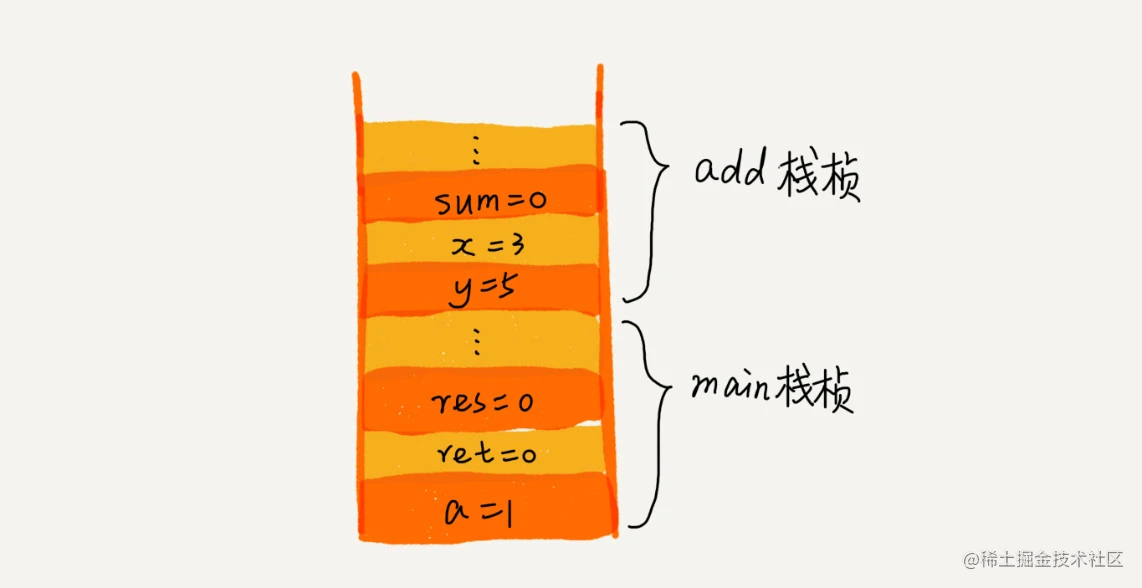# 线性表之栈

## 实现栈

### 数组实现的顺序栈

```java // 基于数组实现的顺序栈 public class ArrayStack { private String[] items; // 数组 private int count; // 栈中元素个数 private int n; //栈的大小

// 初始化数组，申请一个大小为n的数组空间 public ArrayStack(int n) { this.items = new String[n]; this.n = n; this.count = 0; }

// 入栈操作 public boolean push(String item) { // 数组空间不够了，直接返回false，入栈失败。 if (count == n) return false; // 将item放到下标为count的位置，并且count加一 items[count] = item; ++count; return true; }

// 出栈操作 public String pop() { // 栈为空，则直接返回null if (count == 0) return null; // 返回下标为count-1的数组元素，并且栈中元素个数count减一 String tmp = items[count-1]; --count; return tmp; } } ``` 数组的储存空间是必须的，无法省掉，入栈和出栈的空间与时间复杂度O(1)。

## 栈应用

### 函数调用时

```c int main() { int a = 1; int ret = 0; int res = 0; ret = add(3, 5); res = a + ret; printf("%d", res); reuturn 0; }

int add(int x, int y) { int sum = 0; sum = x + y; return sum; } ```# 资料来源

https://time.geekbang.org/column/article/41222